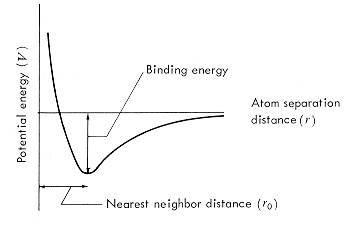0%# 分子动力学是什么？

Molecular dynamics (MD) is a computer simulation method for analyzing the physical movements of atoms and molecules.

# 如何利用分子动力学？

## 势函数（Potential）Ref: https://www.princeton.edu/~maelabs/mae324/glos324/potential.htm

## 化学键作用$E_{bond}$

### 谐振子势（Harmonic）

$$\begin{split} E_{stretch} &= K_{stretch} (r-r_0)^2 \\ E_{bend} &= K_{bend} (\theta-\theta_0)^2 \\ \end{split}$$

In classical mechanics, a harmonic oscillator is a system that, when displaced from its equilibrium position, experiences a restoring force $\vec{F}$ proportional to the displacement $\vec{x}$:

## 非键作用$E_{non-bond}$

### 范德华力

#### 两体势（Pair potential）

$$E^{LJ}_{ij} = 4 \epsilon \left[ \left( \frac{\sigma_{ij}}{r} \right)^{12} - \left( \frac{\sigma_{ij}}{r} \right)^{6} \right]$$

#### 多体势

$$E_{EAM} = \sum_i \left\{ F_i(\rho_i) + \frac{1}{2} \sum_{i \neq j} \phi_{ij} (r_{ij}) \right\}$$

# 参考资料

 The Journal of Chemical Physics 104:21, 8627-8638.

 Daw, Baskes, Phys Rev Lett, 50, 1285 (1983). Daw, Baskes, Phys Rev B, 29, 6443 (1984).